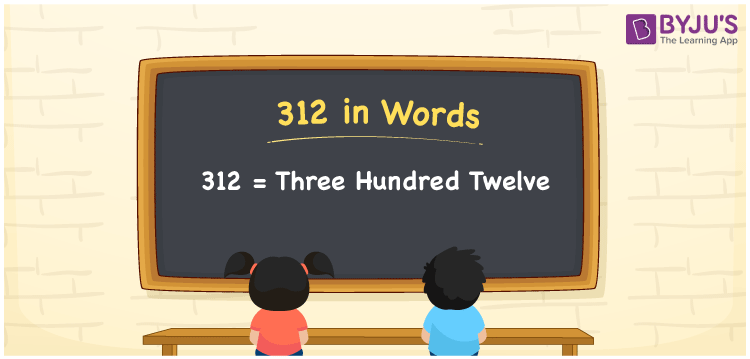# 312 in Words

We can write the number 312 in words as Three hundred twelve. If the sum of three numbers is 312, then you can say, “The sum of three numbers is Three hundred twelve”. That means we use the word form of 312 to communicate the numerical quantity or measure to others. In this article, you will learn how to write the number 312 in words with the help of a place value chart, along with some interesting facts about 312.

 312 in words Three hundred twelve Three hundred twelve in Numbers 312

## 312 in English Words

We generally use the English alphabet to write numbers in words. Thus, we express 312 in English words as “Three hundred twelve”.## How to Write 312 in Words?

The below table represents the place value chart for the number 312. From the below table, we can derive the number 312 in words.

 Hundreds Tens Ones 3 1 2

Here, ones = 2, tens = 1, hundreds = 3

Let us expand the above digits with respect to their place values.

3 × Hundred + 1 × Ten + 2 × One

= 3 × 100 + 1 × 10 + 2 × 1

= 300 + 10 + 2

= Three hundred + Twelve

= Three hundred twelve

Therefore, 312 in words is written as Three hundred twelve.

We know that 312 is a natural number that precedes 313 and succeeds 311.

312 in words – Three hundred twelve

Is 312 an even number? – Yes

Is 312 an odd number? – No

Is 312 a prime number? – No

Is 312 a composite number? – Yes

Is 312 a perfect square number? – No

Is 312 a perfect cube number? – No

## Frequently Asked Questions on 312 in Words

Q1

### How do you write 312 in words?

The number 312 can be written in words as Three hundred twelve.
Q2

### What is the spelling of 312?

The spelling of 312 is used to express or read the number 312, which is “Three hundred twelve”.
Q3

### How to write Rs. 312 in words on a cheque?

On a cheque, we can write the amount Rs. 312 in words as “Three hundred twelve rupees only”.